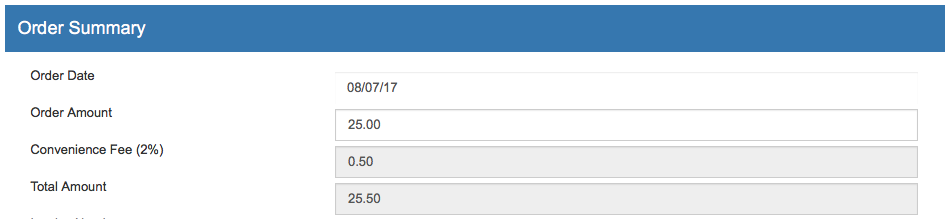Convenience Fees

Certain merchants may want to create a convenience fee or handling charge that is added to the transaction total. We have example code for both flat fees and percentage fees.

This code is for adding a flat fee to each transaction that occurs on your payment form. The below example assumes you are using the default payment form without any changes. Once the form is saved, the customer will be able to type in the amount they are paying and the convenience fee will automatically be added to the transaction total. The customer will not be able to edit the amount of the fee or the grand total of the transaction. An example is shown in the image below:Javascript

Locate this section:

<script type="text/javascript">
var submitted = false;
function submitform()
{
if(submitted) {
return false;
}
submitted=true;
return true;
}
</script>

Insert a new line directly below and add the following code. Please Note: In the line var servicefee = 5 ; the 5 should be replaced with the flat fee you would like to charge. In this example, there is a \$5 flat fee for all transactions:

<script type="text/javascript">
var servicefee = 5 ;
{
var baseamount = document.epayform.baseamount.value ;
var total = (baseamount*1) + servicefee ;
document.epayform.UMamount.value = total ;
console.log(total);
document.getElementById('totalamount').value = total ;
document.getElementById('totalamount').value = CurrencyFormatted(total) ;
}
</script>
<script type="text/javascript">
function CurrencyFormatted(amount)
{
var i = parseFloat(amount);
if(isNaN(i)) { i = 0.00; }
var minus = '';
if(i < 0) { minus = '-'; }
i = Math.abs(i);
i = parseInt((i + .005) * 100);
i = i / 100;
s = new String(i);
if(s.indexOf('.') < 0) { s += '.00'; }
if(s.indexOf('.') == (s.length - 2)) { s += '0'; }
s = minus + s;
return s;
}
</script>

HTML

Next, find the following code in your form:

<div class="form-group">
<span class="col-form-label col-sm-4 col-xs-12 ">Order Amount</span>
<div class="col-md-8 col-xs-12 ">
</div>
</div>

Replace it with the following Please Note: In the Convenience Fee section, replace 5.00 with the correct convenience fee amount:

<div class="form-group">
<span class="col-form-label col-sm-4 col-xs-12 ">Order Amount</span>
<div class="col-md-8 col-xs-12 ">
<input id="" name="baseamount" class="form-control" type="text" onChange="addCharge()" placeholder="Order Amount" value="[UMamount]">
</div>
</div>
<div class="form-group">
<span class="col-form-label col-sm-4 col-xs-12 ">Convenience Fee</span>
<div class="col-md-8 col-xs-12 ">
</div>
</div>
<div class="form-group">
<span class="col-form-label col-sm-4 col-xs-12 ">Total Amount</span>
<div class="col-md-8 col-xs-12 ">
</div>
</div>

Save the changes to your form. Once the form is saved, the customer will be able to type in the amount they are paying and the convenience fee will automatically be added to the transaction total.

Adding a Convenience Fee as a Percentage

This code is for adding a percentage of the transaction that occurs on your payment form. The below example assumes you are using the default payment form without any changes. Once the form is saved, the customer will be able to type in the amount they are paying and the convenience fee will automatically be added to the transaction total. The customer will not be able to edit the amount of the fee or the grand total of the transaction. An example is shown in the image below:Javascript

Locate this section:

<script type="text/javascript">
var submitted = false;
function submitform()
{
if(submitted) {
return false;
}
submitted=true;
return true;
}
</script>

Insert a new line directly below and add the following code. Please Note: In the line var fee = Math.round(amount * .02*100)/100; the .02 should be replaced with the percentage you would like to charge. In this example there is a 2% flat fee for all transactions:

<script type="text/javascript">
function doTotal()
{
var form = document.epayform;
var amount = form.UMcustom1.value = form.UMcustom1.value.replace(/[^0-9\.]/, "");
var fee = Math.round(amount * .02*100)/100;
var total = Math.round(((amount*1)+fee) *100)/100;
form.UMcustom2.value=CurrencyFormatted(fee);
form.UMamount.value=CurrencyFormatted(total);
}
</script>
<script type="text/javascript">
function CurrencyFormatted(amount)
{
var i = parseFloat(amount);
if(isNaN(i)) { i = 0.00; }
var minus = '';
if(i < 0) { minus = '-'; }
i = Math.abs(i);
i = parseInt((i + .005) * 100);
i = i / 100;
s = new String(i);
if(s.indexOf('.') < 0) { s += '.00'; }
if(s.indexOf('.') == (s.length - 2)) { s += '0'; }
s = minus + s;
return s;
}
</script>

fee represents the amount that will be the amount that you are adding on to the transaction.

HTML

Locate the below command in the list of hidden values, and delete it:

<input type="hidden" name="UMamount" value="[UMamount]">

Find the following code in your form:

<div class="form-group">
<span class="col-form-label col-sm-4 col-xs-12 ">Order Amount</span>
<div class="col-md-8 col-xs-12 ">
</div>
</div>

Replace it with the following. Please Note: In the line <span class="col-form-label col-sm-4 col-xs-12 ">Convenience Fee (2%)</span> you should replace the (2%) with the percentage you would like to charge.:

<div class="form-group">
<span class="col-form-label col-sm-4 col-xs-12 ">Order Amount</span>
<div class="col-md-8 col-xs-12 ">
<input id="" name="UMcustom1" class="form-control" type="text" onChange="doTotal()" placeholder="Order Amount" value="[UMcustom1]">
</div>
</div>
<div class="form-group">
<span class="col-form-label col-sm-4 col-xs-12 ">Convenience Fee (2%)</span>
<div class="col-md-8 col-xs-12 ">